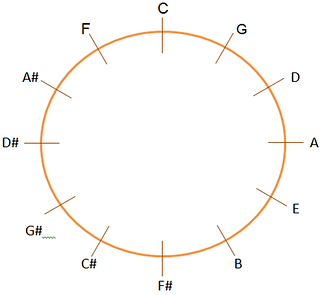The circle of fifths is nothing more than a sequence of notes separated by intervals of perfect fifths.

For example, the sequence: C – G – D – A – E – B is formed by perfect fifth intervals, therefore it is part of a circle of fifths. Notice how B is a fifth above E, that is a fifth above A, and so on. Great, but what’s the use for that?

## How to use Circle of Fifths

Some students learn the circle of fifths to analyze flat and sharp accidents in major scales . Observe:

• The C major scale has no accidents (no scale notes have sharps or flats).
• The G note is a fifth above C, and the scale of G major has one accident, the F# note.
• The D note is a fifth above G, and the scale of D major has two accidents, the (the F# and C# notes).

Moral of the story: On every fifth, there is one more accident on the next scale. This is especially useful for keyboard players, as each major scale on the keyboard has a different shape, and the amount of accidents will define how many black keys the scale will have. See a table with the accidents for each major scale below:Okay, but this is not the only use for the circle of fifths. It is interesting to note that the dominant V7 chords can be “stacked” one after the other, forming a sequence of resolutions based on fifths. When this occurs, these dominants are called “extended dominants“.  For example:

| A7 | D7 | G7 | C |

Notice how, in this sequence, the A7 chord resolved in its tonic (D), but this D did not have the tonic function, but a dominant one, resolving in G. Likewise, G did not act as a tonic, but as a dominant that resolved in C. Therefore, we had a sequence of extended dominants; and observing from right to left, the fifth degree of C is G, the fifth degree of G is D, and so on. That is, we form a circle of fifths.

## The relationship between the Circle of Fifths and the Pentatonic Scale

Not everyone knows, but the pentatonic scale arises from a circle of fifths! Observe the sequence C – G – D – A – E (circle of fifths starting from the C note). Now compare with the notes of the C major pentatonic scale: C, D, E, G, A.

As we can see, when we take the first 5 notes of a circle of fifths, we are forming a pentatonic scale.

Okay, so far we’ve only talked about the circle of fifths; What about the circle of fourths?

## The Circle of Fourths

The circle of fourths is nothing more than a circle of fifths seen in reverse. Notice the previous sequence:

C – G – D – A – E

This sequence seen from left to right has fifth intervals. The same sequence, when seen from right to left, has fourth intervals. In other words, the circle of fifths is the inverted cycle of fourths and vice versa.

## The complete circle of fifths

Ok, so let’s finish this topic by drawing the complete circle of fifths. We will make a circle and put all 12 notes spaced by fifth intervals:Note that, clockwise, we have the circle of fifths and, counterclockwise, we have the cycle of fourths.

Go to: Secondary Dominants

Back to: Module 7